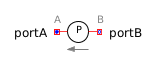Lim Integrator - MapleSim Help

Limited Integrator

Integrator with limited valueDescription The Limited Integrator (or Lim Integrator) component computes, element-wise, the integral of the input $u$ and then multiplies it by the gain, $k$. If the output exceeds the specified upper or lower limit, the integration is stopped and is restarted when the input drives the integral away from the limit. The Signal Size parameter assigns the dimension of the input and output connectors.Equations ${\stackrel{.}{y}}_{j}=\left\{\begin{array}{cc}k{u}_{j}& {\mathrm{out}}_{\mathrm{min}}Connections

 Name Description Modelica ID $u$ Real input vector u $y$ Real output vector y $\mathrm{reset}$ Optional connector of reset signal reset $\mathrm{set}$ Optional connector of set signal setParameters

 Name Default Units Description Modelica ID Signal Size $1$ Dimension of input and output signals signalSize k $1$ Integrator gain k ${\mathrm{out}}_{\mathrm{max}}$ $1$ Upper limit of output outMax ${\mathrm{out}}_{\mathrm{min}}$ $-\mathrm{outMax}$ Lower limit of output outMin use reset $\mathrm{false}$ True(checked) enables reset port use_reset use set $\mathrm{false}$ True(checked) enables port that sets reset value (when use reset is selected) use_set Strict $\mathrm{false}$ True (checked) means no event is generated when the output reaches a limit strict Initial Values Initialization with initial states Type of initialization initType Limits At Initial $\mathrm{true}$ True (checked) means limits are used during initialization limitsAtInit ${y}_{0}$ $0$ Initial or guess value of the output. This value must be within the limits y_startModelica Standard Library The component described in this topic is from the Modelica Standard Library. To view the original documentation, which includes author and copyright information, click here.